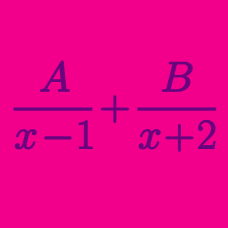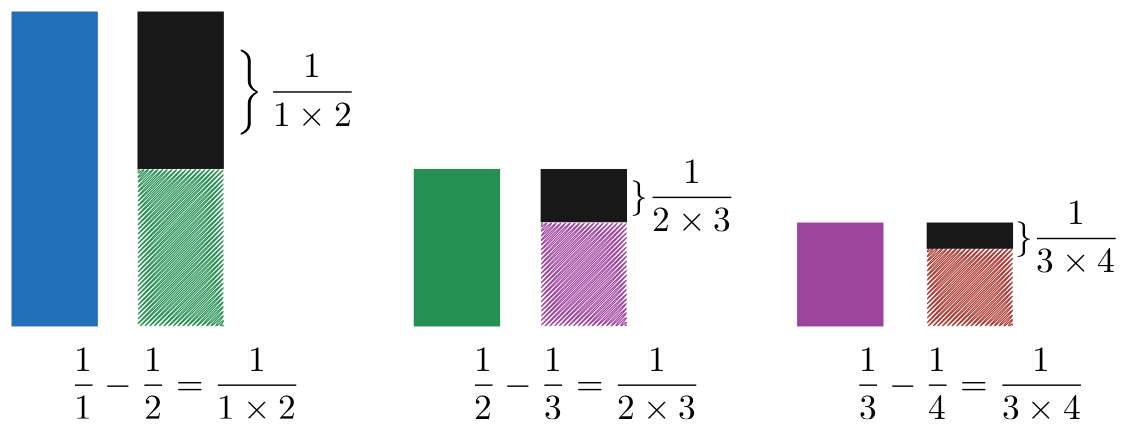Algebra

# Partial Fractions: Level 2 Challenges

$\large \displaystyle \sum_{n = 1}^{\infty} \dfrac {1}{n^2 + 3n + 2} = \ ?$

$1 + \frac {1}{\color{#3D99F6}2} + \frac {1}{\color{#3D99F6}6} + \frac {1}{\color{#3D99F6}{12}} + \frac {1}{\color{#3D99F6}{20}} + \ldots = \ \color{teal}?$Consider the following pattern:

\begin{aligned} \frac{1}{1\times 2} & = \frac{1}{1} - \frac{1}{2} \\ & \\ \frac{1}{2\times 3} & = \frac{1}{2} - \frac{1}{3} \\ & \\ \frac{1}{3\times 4} & = \frac{1}{3} - \frac{1}{4} \\ \vdots & \end{aligned}

Following the pattern above, if $\displaystyle \frac{1}{11\times 12} = \frac{1}{a} - \frac{1}{b},$ what are the values of $a$ and $b$?

$\large \frac{1}{1 \times 2}+\frac{1}{2 \times 3}+\frac{1}{3 \times 4}+\cdots +\frac{1}{99 \times 100} = \ ?$

$\large \frac{1}{1\cdot 3} + \frac{1}{3\cdot 5} +\frac{1}{5\cdot 7} + \frac{1}{7 \cdot 9} + \cdots = \ ?$

×